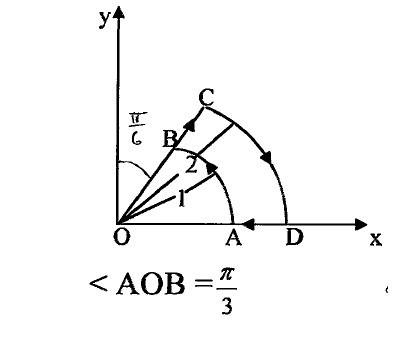# Stoke's Theorem vector field

xxsteelxx

## Homework Statement

Assume a vector field:$$\textbf{F} = \widehat{r} 2r sin\phi + \widehat{\phi} r^2 cos\phi$$

a) Verify the Stokes's theorem over the ABCD contour shown in Fig. 1 .
b) Can F be expressed as the gradient of a scalar? ExplainMy problems results in not being able to verify Stoke's Theorem

## Homework Equations

Stoke's Theorem
$$\oint_{C} \textbf{F}\cdot \overrightarrow{dl} = \int \int_{S} (\nabla\times\textbf{F})\cdot \overrightarrow{dS}$$

## The Attempt at a Solution

We can see that the line integral and surface integrals can be dealt with in cylindrical coordinates.
for line integrals:
$$\int _{DA}+\int _{AB}+\int _{BC}+\int _{CD} =-\int_{r=2}^{1}2rsin(0)dr + \int_{\phi=0}^{\pi/3}cos(\phi)d\phi + \int_{r=1}^{2}2rsin(\pi/3)dr + \int_{\phi=\pi/3}^{0}4cos(\phi)d\phi$$
$$= \frac{\sqrt3}{2} +\frac{\sqrt3}{2}(4-1) -4\frac{\sqrt3}{2}=0$$

I also obtain that $$\nabla\cdot \textbf{F}=\widehat{z}cos\phi(3r-2)$$
And surface integral is evaluated as $$-\int_{r=1}^{2}\int _{\phi=0}^{\phi/3}cos\phi(3r-2)rdrd\phi=-(8-4)\frac{\sqrt3}{2}=-4\frac{\sqrt3}{2}$$
The negative is due to the fact that ds is in the -z direction.
Am I doing something wrong while integrating?

Staff Emeritus
Homework Helper
In your line integrals for segments AB and CD, d$\phi$ represents a change in angle, which s not the same as a change in arc length, dl.

•1 person
xxsteelxx
True, but isn't $$\overrightarrow{dl} = \widehat{\phi}r d\phi$$ for path AB and $$\overrightarrow{dl} = -\widehat{\phi} r d\phi$$ for path CD? The after dot product, I obtain the integrals previously?

SteamKings point is that, in the integral from C to D, $ds= 2d\phi$. You need another factor of 2.
•